# 1 Introduction

This vignette will demonstrate a full single-cell lineage analysis workflow, with particular emphasis on the processes of lineage reconstruction and pseudotime inference. We will make use of the slingshot package proposed in (Street et al. 2017) and show how it may be applied in a broad range of settings.

The goal of slingshot is to use clusters of cells to uncover global structure and convert this structure into smooth lineages represented by one-dimensional variables, called “pseudotime.” We provide tools for learning cluster relationships in an unsupervised or semi-supervised manner and constructing smooth curves representing each lineage, along with visualization methods for each step.

## 1.1 Overview

The minimal input to slingshot is a matrix representing the cells in a reduced-dimensional space and a vector of cluster labels. With these two inputs, we then:

• Identify the global lineage structure by constructing an minimum spanning tree (MST) on the clusters, with the getLineages function.
• Construct smooth lineages and infer pseudotime variables by fitting simultaneous principal curves with the getCurves function.
• Assess the output of each step with built-in visualization tools.

## 1.2 Datasets

We will work with two simulated datasets in this vignette. The first (referred to as the “single-trajectory” dataset) is generated below and designed to represent a single lineage in which one third of the genes are associated with the transition. This dataset will be contained in a SingleCellExperiment object (Lun and Risso 2017) and will be used to demonstrate a full “start-to-finish” workflow.

# generate synthetic count data representing a single lineage
means <- rbind(
# non-DE genes
matrix(rep(rep(c(0.1,0.5,1,2,3), each = 300),100),
ncol = 300, byrow = TRUE),
# early deactivation
matrix(rep(exp(atan( ((300:1)-200)/50 )),50), ncol = 300, byrow = TRUE),
# late deactivation
matrix(rep(exp(atan( ((300:1)-100)/50 )),50), ncol = 300, byrow = TRUE),
# early activation
matrix(rep(exp(atan( ((1:300)-100)/50 )),50), ncol = 300, byrow = TRUE),
# late activation
matrix(rep(exp(atan( ((1:300)-200)/50 )),50), ncol = 300, byrow = TRUE),
# transient
matrix(rep(exp(atan( c((1:100)/33, rep(3,100), (100:1)/33) )),50),
ncol = 300, byrow = TRUE)
)
counts <- apply(means,2,function(cell_means){
total <- rnbinom(1, mu = 7500, size = 4)
rmultinom(1, total, cell_means)
})
rownames(counts) <- paste0('G',1:750)
colnames(counts) <- paste0('c',1:300)
sim <- SingleCellExperiment(assays = List(counts = counts))

The second dataset (the “bifurcating” dataset) consists of a matrix of coordinates (as if obtained by PCA, ICA, diffusion maps, etc.) along with cluster labels generated by $$k$$-means clustering. This dataset represents a bifurcating trajectory and it will allow us to demonstrate some of the additional functionality offered by slingshot.

library(slingshot, quietly = FALSE)
data("slingshotExample")
rd <- slingshotExample$rd cl <- slingshotExample$cl

dim(rd) # data representing cells in a reduced dimensional space
##  140   2
length(cl) # vector of cluster labels
##  140

# 2 Upstream Analysis

## 2.1 Gene Filtering

To begin our analysis of the single lineage dataset, we need to reduce the dimensionality of our data and filtering out uninformative genes is a typical first step. This will greatly improve the speed of downstream analyses, while keeping the loss of information to a minimum.

For the gene filtering step, we retained any genes robustly expressed in at least enough cells to constitute a cluster, making them potentially interesting cell-type marker genes. We set this minimum cluster size to 10 cells and define a gene as being “robustly expressed” if it has a simulated count of at least 3 reads.

# filter genes down to potential cell-type markers
# at least M (15) reads in at least N (15) cells
geneFilter <- apply(assays(sim)$counts,1,function(x){ sum(x >= 3) >= 10 }) sim <- sim[geneFilter, ] ## 2.2 Normalization Another important early step in most RNA-Seq analysis pipelines is the choice of normalization method. This allows us to remove unwanted technical or biological artifacts from the data, such as batch, sequencing depth, cell cycle effects, etc. In practice, it is valuable to compare a variety of normalization techniques and compare them along different evaluation criteria, for which we recommend the scone package (Cole and Risso 2018). We also note that the order of these steps may change depending on the choice of method. ZINB-WaVE (Risso et al. 2018) performs dimensionality reduction while accounting for technical variables and MNN (Haghverdi et al. 2018) corrects for batch effects after dimensionality reduction. Since we are working with simulated data, we do not need to worry about batch effects or other potential confounders. Hence, we will proceed with full quantile normalization, a well-established method which forces each cell to have the same distribution of expression values. FQnorm <- function(counts){ rk <- apply(counts,2,rank,ties.method='min') counts.sort <- apply(counts,2,sort) refdist <- apply(counts.sort,1,median) norm <- apply(rk,2,function(r){ refdist[r] }) rownames(norm) <- rownames(counts) return(norm) } assays(sim)$norm <- FQnorm(assays(sim)$counts) ## 2.3 Dimensionality Reduction The fundamental assumption of slingshot is that cells which are transcriptionally similar will be close to each other in some reduced-dimensional space. Since we use Euclidean distances in constructing lineages and measuring pseudotime, it is important to have a low-dimensional representation of the data. There are many methods available for this task and we will intentionally avoid the issue of determining which is the “best” method, as this likely depends on the type of data, method of collection, upstream computational choices, and many other factors. We will demonstrate two methods of dimensionality reduction: principal components analysis (PCA) and diffusion maps (via the destiny package, (Angerer et al. 2015)). When performing PCA, we do not scale the genes by their variance because we do not believe that all genes are equally informative. We want to find signal in the robustly expressed, highly variable genes, not dampen this signal by forcing equal variance across genes. When plotting, we make sure to set the aspect ratio, so as not to distort the perceived distances. pca <- prcomp(t(log1p(assays(sim)$norm)), scale. = FALSE)
rd1 <- pca$x[,1:2] plot(rd1, col = rgb(0,0,0,.5), pch=16, asp = 1)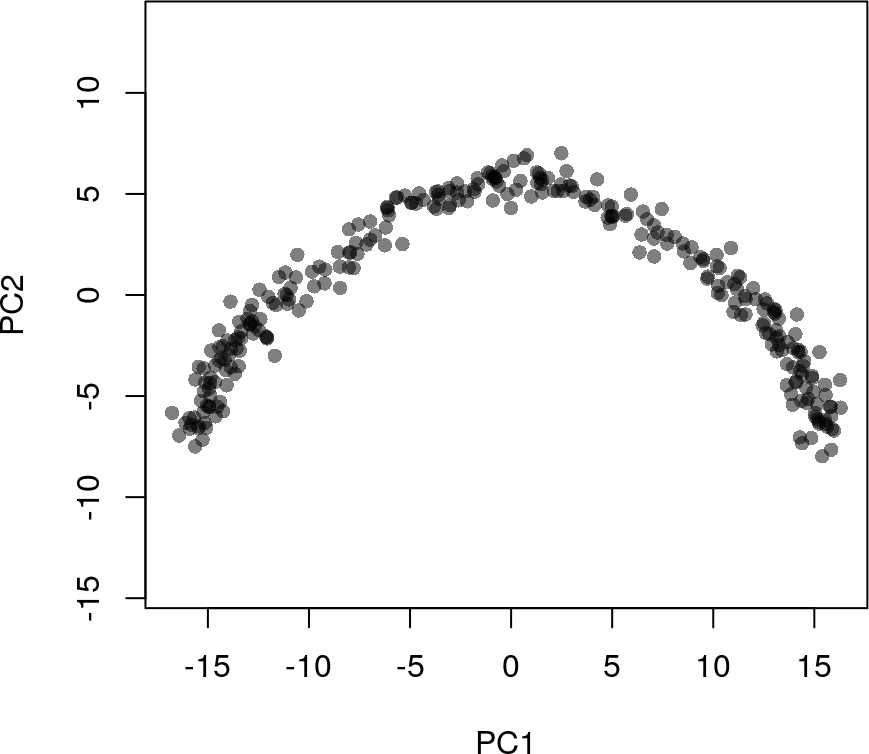library(destiny, quietly = TRUE) ## ## Attaching package: 'destiny' ## The following object is masked from 'package:SummarizedExperiment': ## ## distance ## The following object is masked from 'package:GenomicRanges': ## ## distance ## The following object is masked from 'package:IRanges': ## ## distance dm <- DiffusionMap(t(log1p(assays(sim)$norm)))
## Warning in DiffusionMap(t(log1p(assays(sim)$norm))): You have 724 genes. ## Consider passing e.g. n_pcs = 50 to speed up computation. rd2 <- cbind(DC1 = dm$DC1, DC2 = dm$DC2) plot(rd2, col = rgb(0,0,0,.5), pch=16, asp = 1)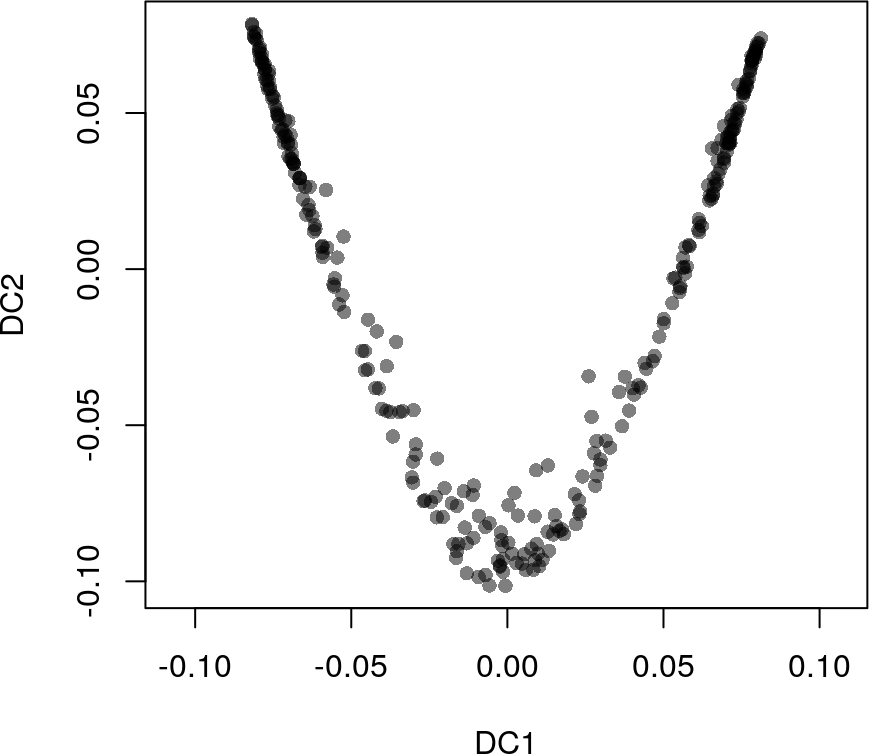We will add both dimensionality reductions to the SingleCellExperiment object, but continue our analysis focusing on the PCA results. reducedDims(sim) <- SimpleList(PCA = rd1, DiffMap = rd2) ## 2.4 Clustering Cells The final input to slingshot is a vector of cluster labels for the cells. If this is not provided, slingshot will treat the data as a single cluster and fit a standard principal curve. However, we recommend clustering the cells even in datasets where only a single lineage is expected, as it allows for the potential discovery of novel branching events. The clusters identified in this step will be used to determine the global structure of the underlying lineages (that is, their number, when they branch off from one another, and the approximate locations of those branching events). This is different than the typical goal of clustering single-cell data, which is to identify all biologically relevant cell types present in the dataset. For example, when determining global lineage structure, there is no need to distinguish between immature and mature neurons since both cell types will, presumably, fall along the same segment of a lineage. For our analysis, we implement two clustering methods which similarly assume that Euclidean distance in a low-dimensional space reflect biological differences between cells: Gaussian mixture modeling and $$k$$-means. The former is implemented in the mclust package (Scrucca et al. 2016) and features an automated method for determining the number of clusters based on the Bayesian information criterion (BIC). library(mclust, quietly = TRUE) ## Package 'mclust' version 5.4.6 ## Type 'citation("mclust")' for citing this R package in publications. ## ## Attaching package: 'mclust' ## The following object is masked from 'package:mgcv': ## ## mvn cl1 <- Mclust(rd1)$classification
colData(sim)$GMM <- cl1 library(RColorBrewer) plot(rd1, col = brewer.pal(9,"Set1")[cl1], pch=16, asp = 1)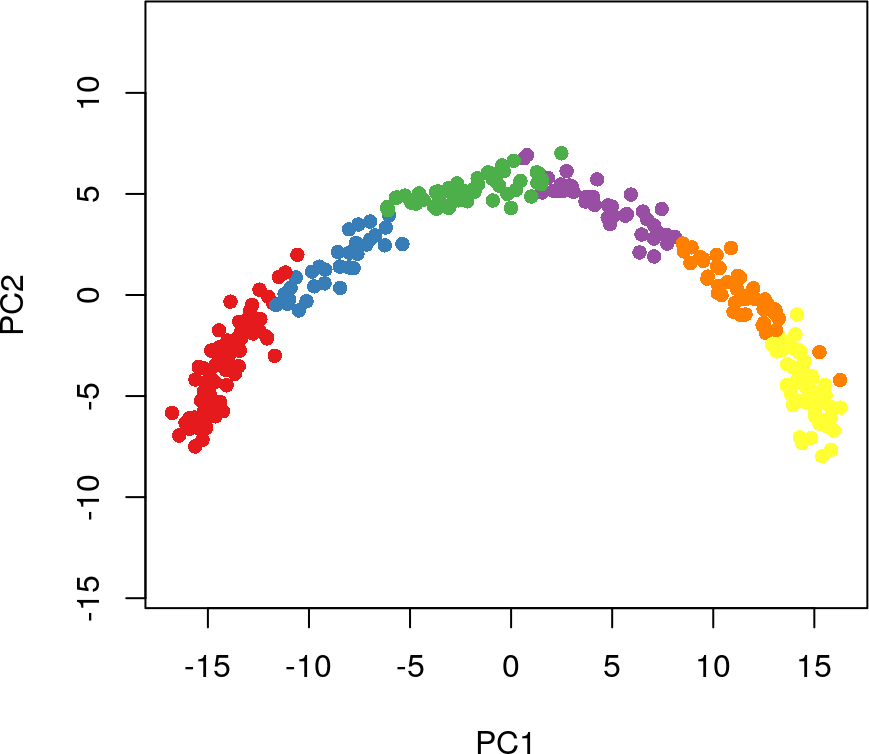While $$k$$-means does not have a similar functionality, we have shown in (Street et al. 2017) that simultaneous principal curves are quite robust to the choice of $$k$$, so we select a $$k$$ of 4 somewhat arbitrarily. If this is too low, we may miss a true branching event and if it is too high or there is an abundance of small clusters, we may begin to see spurious branching events. cl2 <- kmeans(rd1, centers = 4)$cluster
colData(sim)$kmeans <- cl2 plot(rd1, col = brewer.pal(9,"Set1")[cl2], pch=16, asp = 1)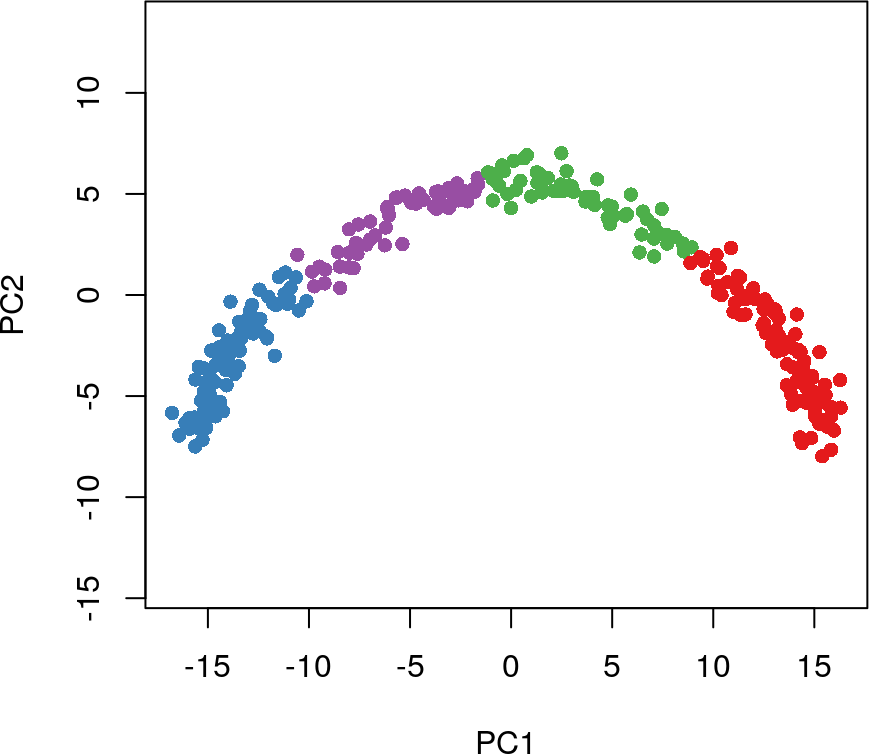# 3 Using Slingshot At this point, we have everything we need to run slingshot on our simulated dataset. This is a two-step process composed of identifying the global lineage structure with a cluster-based minimum spanning tree (MST) and fitting simultaneous principal curves to describe each lineage. These two steps can be run separately with the getLineages and getCurves functions, or together with the wrapper function, slingshot (recommended). We will use the wrapper function for the analysis of the single-trajectory dataset, but demonstrate the usage of the individual functions later, on the bifurcating dataset. The slingshot wrapper function performs both steps of trajectory inference in a single call. The necessary inputs are a reduced dimensional matrix of coordinates and a set of cluster labels. These can be separate objects or, in the case of the single-trajectory data, elements contained in a SingleCellExperiment object. To run slingshot with the dimensionality reduction produced by PCA and cluster labels identified by Gaussian mixutre modeling, we would do the following: sim <- slingshot(sim, clusterLabels = 'GMM', reducedDim = 'PCA') ## Using full covariance matrix ## Warning in if (class(X) == "dist") X <- as.matrix(X): the condition has length > ## 1 and only the first element will be used As noted above, if no clustering results are provided, it is assumed that all cells are part of the same cluster and a single curve will be constructed. If no dimensionality reduction is provided, slingshot will use the first element of the list returned by reducedDims. The output is a SingleCellExperiment object with slingshot results incorporated. Most of the output is added to the metadata in the form of a list and is accessible via metadata(sce)$slingshot. Additionally, all inferred pseudotime variables (one per lineage) are added to the colData. To extract all slingshot results in a single object, we can use the SlingshotDataSet function. This can be useful for visualization, as several plotting methods for SlingshotDataSet objects are included with the package. Below, we visuzalize the inferred lineage for the single-trajectory data with points colored by pseudotime.

summary(sim$slingPseudotime_1) ## Min. 1st Qu. Median Mean 3rd Qu. Max. ## 0.000 8.633 21.118 21.415 34.367 43.186 colors <- colorRampPalette(brewer.pal(11,'Spectral')[-6])(100) plotcol <- colors[cut(sim$slingPseudotime_1, breaks=100)]

plot(reducedDims(sim)$PCA, col = plotcol, pch=16, asp = 1) lines(SlingshotDataSet(sim), lwd=2, col='black')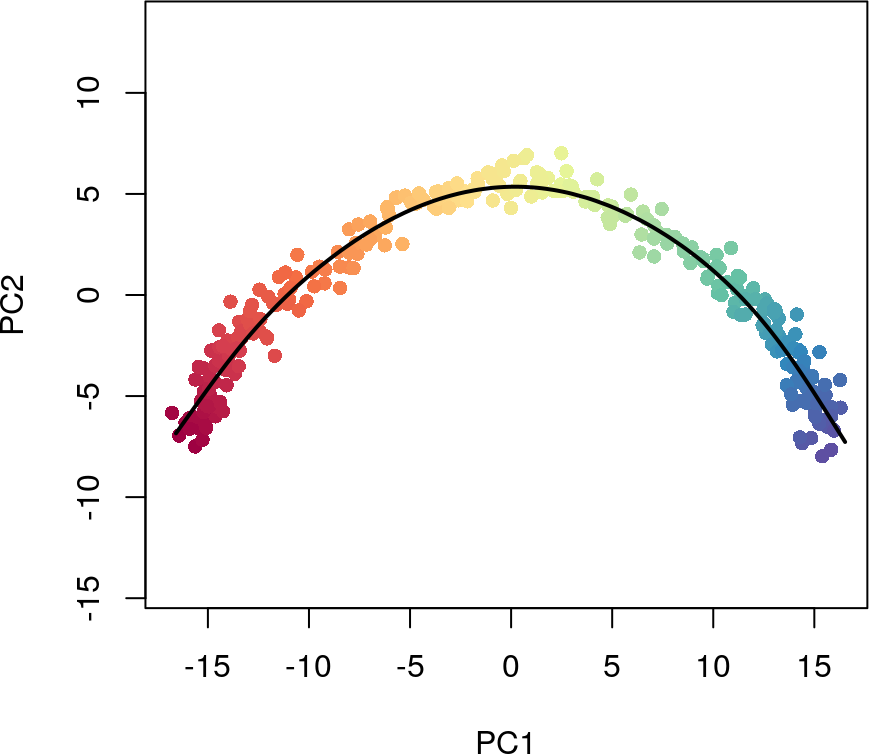We can also see how the lineage structure was intially estimated by the cluster-based minimum spanning tree by using the type argument. plot(reducedDims(sim)$PCA, col = brewer.pal(9,'Set1')[sim$GMM], pch=16, asp = 1) lines(SlingshotDataSet(sim), lwd=2, type = 'lineages', col = 'black')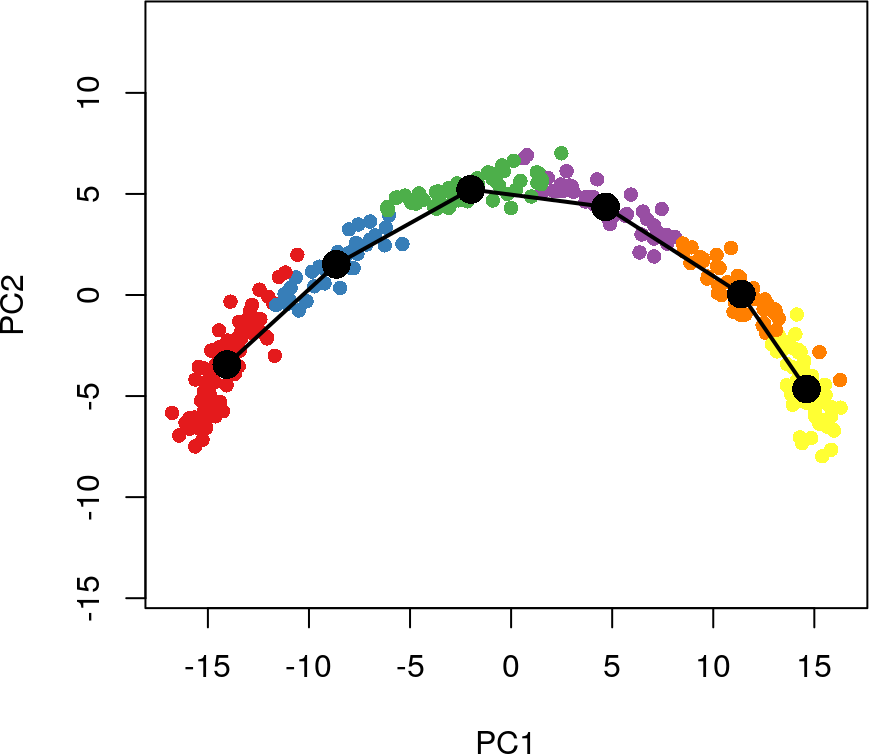# 4 Downstream Analysis ## 4.1 Identifying temporally expressed genes After running slingshot, an interesting next step may be to find genes that change their expression over the course of development. We demonstrate one possible method for this type of analysis on the $$100$$ most variable genes. We will regress each gene on the pseudotime variable we have generated, using a general additive model (GAM). This allows us to detect non-linear patterns in gene expression. require(gam) ## Loading required package: gam ## Loading required package: splines ## Loading required package: foreach ## Loaded gam 1.16.1 ## ## Attaching package: 'gam' ## The following objects are masked from 'package:mgcv': ## ## gam, gam.control, gam.fit, s t <- sim$slingPseudotime_1

# for time, only look at the 100 most variable genes
Y <- log1p(assays(sim)$norm) var100 <- names(sort(apply(Y,1,var),decreasing = TRUE))[1:100] Y <- Y[var100,] # fit a GAM with a loess term for pseudotime gam.pval <- apply(Y,1,function(z){ d <- data.frame(z=z, t=t) suppressWarnings({ tmp <- suppressWarnings(gam(z ~ lo(t), data=d)) }) p <- summary(tmp)[][2,3] p }) We can then pick out the top genes based on p-value and visualize their expression over developmental time with a heatmap. topgenes <- names(sort(gam.pval, decreasing = FALSE))[1:100] heatdata <- assays(sim)$norm[topgenes, order(t, na.last = NA)]
heatclus <- sim\$GMM[order(t, na.last = NA)]

heatmap(log1p(heatdata), Colv = NA,
ColSideColors = brewer.pal(9,"Set1")[heatclus])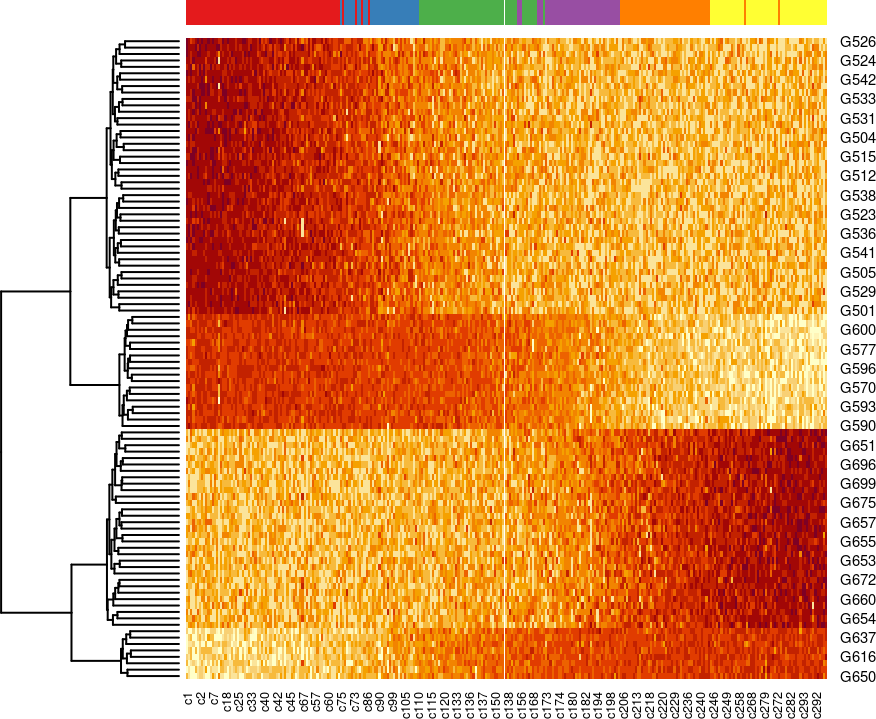# 5 Detailed Slingshot Functionality

Here, we provide further details and highlight some additional functionality of the slingshot package. We will use the included slingshotExample dataset for illustrative purposes. This dataset was designed to represent cells in a low dimensional space and comes with a set of cluster labels generated by $$k$$-means clustering. Rather than constructing a full SingleCellExperiment object, which requires gene-level data, we will use the low-dimensional matrix of coordinates directly and provide the cluster labels as an additional argument.

## 5.1 Identifying global lineage structure

The getLineages function takes as input an n $$\times$$ p matrix and a vector of clustering results of length n. It maps connections between adjacent clusters using a minimum spanning tree (MST) and identifies paths through these connections that represent lineages. The output of this function is a SlingshotDataSet containing the inputs as well as the inferred MST (represented by an adjacency matrix) and lineages (ordered vectors of cluster names).

This analysis can be performed in an entirely unsupervised manner or in a semi-supervised manner by specifying known initial and terminal point clusters. If we do not specify a starting point, slingshot selects one based on parsimony, maximizing the number of clusters shared between lineages before a split. If there are no splits or multiple clusters produce the same parsimony score, the starting cluster is chosen arbitrarily.

In the case of our simulated data, slingshot selects Cluster 1 as the starting cluster. However, we generally recommend the specification of an initial cluster based on prior knowledge (either time of sample collection or established gene markers). This specification will have no effect on how the MST is constructed, but it will impact how branching curves are constructed.

lin1 <- getLineages(rd, cl, start.clus = '1')
## Using full covariance matrix
## Warning in if (class(X) == "dist") X <- as.matrix(X): the condition has length >
## 1 and only the first element will be used
lin1
## class: SlingshotDataSet
##
##  Samples Dimensions
##      140          2
##
## lineages: 2
## Lineage1: 1  2  3  5
## Lineage2: 1  2  3  4
##
## curves: 0
plot(rd, col = brewer.pal(9,"Set1")[cl], asp = 1, pch = 16)
lines(lin1, lwd = 3, col = 'black')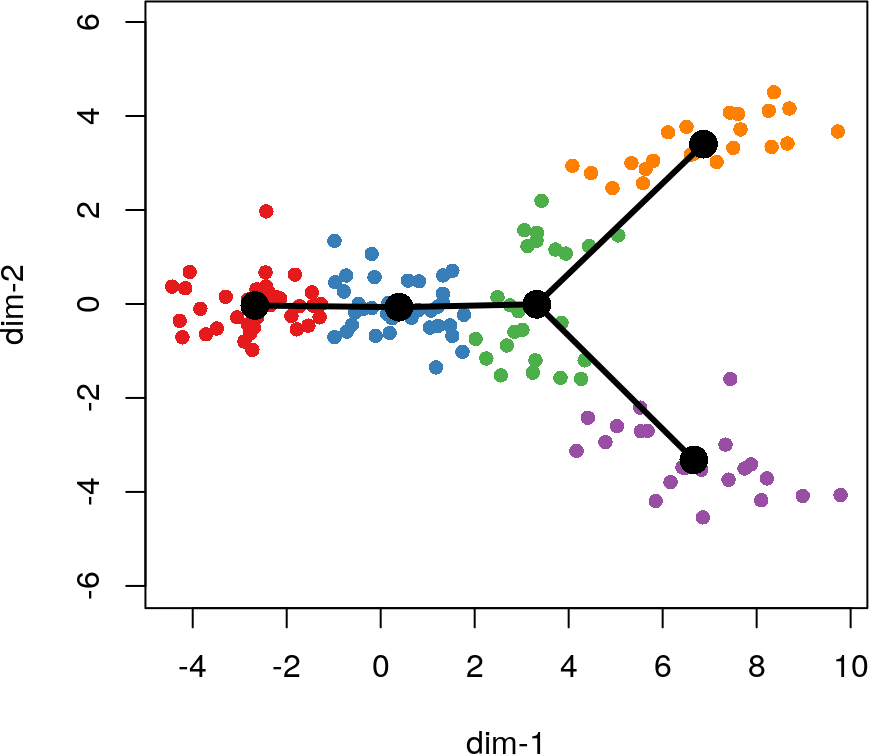At this step, slingshot also allows for the specification of known endpoints. Clusters which are specified as terminal cell states will be constrained to have only one connection when the MST is constructed (ie., they must be leaf nodes). This constraint could potentially impact how other parts of the tree are drawn, as shown in the next example where we specify Cluster 3 as an endpoint.

lin2 <- getLineages(rd, cl, start.clus= '1', end.clus = '3')
## Using full covariance matrix
## Warning in if (class(X) == "dist") X <- as.matrix(X): the condition has length >
## 1 and only the first element will be used
plot(rd, col = brewer.pal(9,"Set1")[cl], asp = 1, pch = 16)
lines(lin2, lwd = 3, col = 'black', show.constraints = TRUE)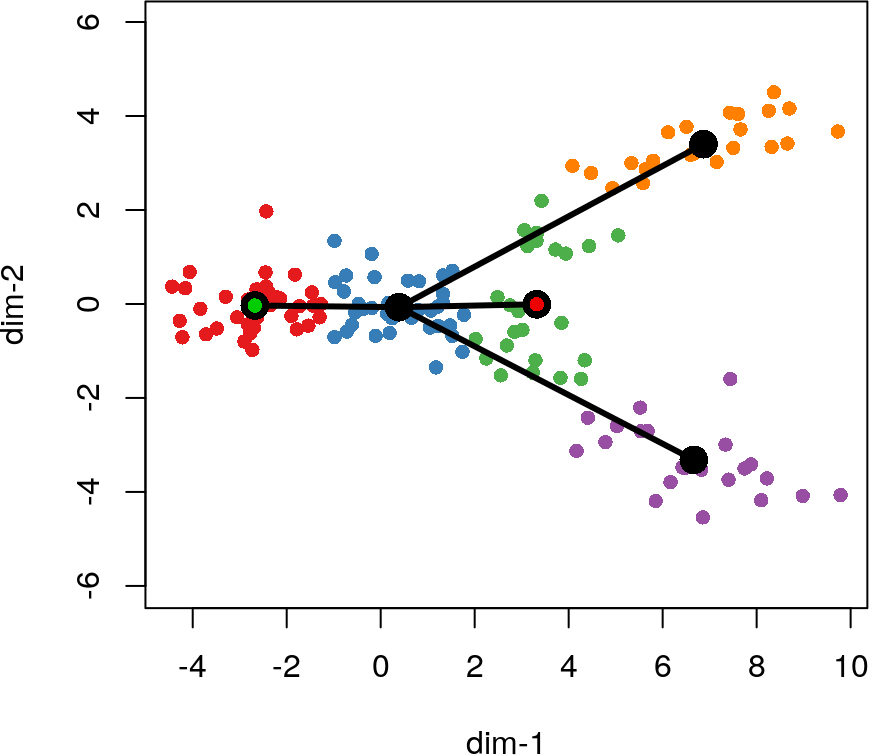This type of supervision can be useful for ensuring that results are consistent with prior biological knowledge. Specifically, it can prevent known terminal cell fates from being categorized as transitory states.

There are a few additional arguments we could have passed to getLineages for greater control:

• dist.fun is a function for computing distances between clusters. The default is squared distance between cluster centers normalized by their joint covariance matrix.
• omega is a granularity parameter, allowing the user to set an upper limit on connection distances. This can be useful for identifying outlier clusters which are not a part of any lineage.

After constructing the MST, getLineages identifies paths through the tree to designate as lineages. At this stage, a lineage will consist of an ordered set of cluster names, starting with the root cluster and ending with a leaf. The output of getLineages is a SlingshotDataSet which holds all of the inputs as well as a list of lineages and some additional information on how they were constructed. This object is then used as the input to the getCurves function.

## 5.2 Constructing smooth curves and ordering cells

In order to model development along these various lineages, we will construct smooth curves with the function getCurves. Using smooth curves based on all the cells eliminates the problem of cells projecting onto vertices of piece-wise linear trajectories and makes slingshot more robust to noise in the clustering results.

In order to construct smooth lineages, getCurves follows an iterative process similar to that of principal curves presented in (Hastie and Stuetzle 1989). When there is only a single lineage, the resulting curve is simply the principal curve through the center of the data, with one adjustment: the initial curve is constructed with the linear connections between cluster centers rather than the first prinicpal component of the data. This adjustment adds stability and typically hastens the algorithm’s convergence.

When there are two or more lineages, we add an additional step to the algorithm: averaging curves near shared cells. Both lineages should agree fairly well on cells that have yet to differentiate, so at each iteration we average the curves in the neighborhood of these cells. This increases the stability of the algorithm and produces smooth branching lineages.

crv1 <- getCurves(lin1)
crv1
## class: SlingshotDataSet
##
##  Samples Dimensions
##      140          2
##
## lineages: 2
## Lineage1: 1  2  3  5
## Lineage2: 1  2  3  4
##
## curves: 2
## Curve1: Length: 15.045   Samples: 100.6
## Curve2: Length: 15.126   Samples: 103.5
plot(rd, col = brewer.pal(9,"Set1")[cl], asp = 1, pch = 16)
lines(crv1, lwd = 3, col = 'black')